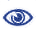# Prague Stringology Conference 2013

The Sum of Exponents of Maximal Repetitions in Standard Sturmian Words

 Abstract: A maximal repetition is a non-extendable (with the same period) periodic segment in a string, in which the period repeats at least twice. In this paper we study problems related to the structure of maximal repetitions in standard Sturmian words and present the formulas for the sum of their exponents. Moreover, we show how to compute the sum of exponents of maximal repetitions in any standard Sturmian word in linear time with respect to the (total) size of its compressed representation. The presented formulas and algorithm can be easily modified to obtain the total run length of the word.

 Download paper:PDF BibTeX reference
 Download presentation: